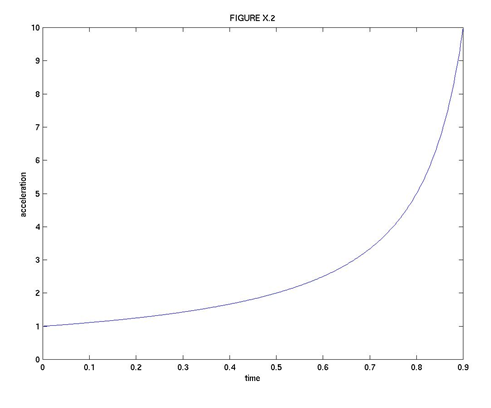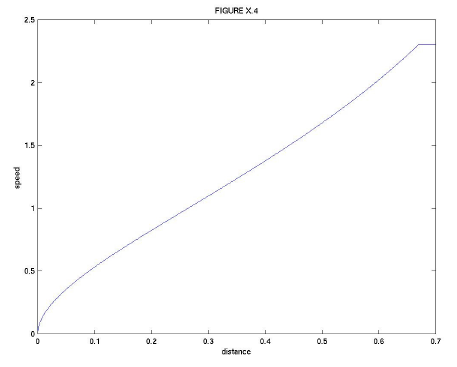$$\require{cancel}$$

10.3: The Rocket Equation

•• Contributed by Jeremy Tatum
• Emeritus Professor (Physics & Astronomy) at University of Victoria

Initially at time $$t$$ = 0, the mass of the rocket, including fuel, is $$m_{0}$$.

We suppose that the rocket is burning fuel at a rate of $$b$$ kg s-1 so that, at time $$t$$, the mass of the rocket-plus-remaining-fuel is $$m=m_{0}-bt$$. The rate of increase of mass with time is $$\frac{dm}{dt}=-b$$ and is supposed constant with time. (The rate of "increase" is, of course, negative.)

We suppose that the speed of the ejected fuel, relative to the rocket, is $$V$$. The thrust of the ejected fuel on the rocket is therefore $$Vb$$, or $$-V\frac{dm}{dt}$$. This is equal to the instantaneous mass times acceleration of the rocket:

$Vb=m\frac{dv}{dt}=(m_{0}-bt)\frac{dv}{dt}. \label{10.3.1}$

Thus

$\int_{0}^{v}dv=Vb\int_{0}^{t}\frac{dt}{m_{0}-bt}. \label{10.3.2}$

(Don't be tempted to write the right hand side as $$-Vb\int_{0}^{t}\frac{dt}{bt-m_{0}}$$. You are anticipating a logarithm, so keep the denominator positive. We have met this before in Chapter 6.) On integration, we obtain

$v=V\ln\frac{m_{0}}{m_{0}-bt}. \label{10.3.3}$

The acceleration is

$\frac{dv}{dt}=\frac{Vb}{m_{0}-bt}. \label{10.3.4}$

At $$t$$ = 0, the speed is zero and the acceleration is $$\frac{Vb}{m_{0}}$$.

At time $$t=\frac{m_{0}}{b}$$, the remaining mass is zero and the speed and acceleration are both infinite. However, this is so only if the initial mass is 100% fuel and nothing else. This is not realistic. If the fraction of the total mass was initially $$f$$, the fuel will be completely expended after a time $$\frac{fm_{0}}{b}$$ at which time the speed will be (which is, of course, positive), and the speed will remain constant thereafter. For example, if $$f$$ = 99%, the final speed will be 4.6 $$V$$.

Equations $$\ref{10.3.3}$$ and $$\ref{10.3.4}$$ are shown in Figures X.1 and X.2. In Figure X.1, the speed of the rocket is plotted in units of $$V$$, the ejection speed of the burnt fuel. The time is plotted in units of $$\frac{m_{0}}{b}$$. The fuel initially comprised 90% of the rocket, so that the rocket runs out of fuel in time 0.9 $$\frac{m_{0}}{b}$$, at which time its speed is 2.3$$V$$. In Figure X.2, the acceleration is plotted in units of the initial acceleration, which is $$\frac{Vb}{m_{0}}$$. When the fuel is exhausted, the acceleration is ten times this.In Equation $$\ref{10.3.3}$$, $$v$$ is of course $$\frac{dx}{dt}$$, so the equation can be integrated to obtain the distance:time relation:

$x=V[t+(\frac{m_{0}}{b}-t)\ln(1-\frac{bt}{m_{0}})]. \label{10.3.5}$

Elimination of $$t$$ between Equations $$\ref{10.3.3}$$ and $$\ref{10.3.5}$$ gives the relation between speed and distance:

$x=\frac{Vm_{0}}{b}[1-(1+\frac{v}{V})e^{\frac{-v}{V}}]. \label{10.3.6}$

If $$f$$ is the fraction of the initial mass that is fuel, the fuel supply will be exhausted after a time $$\frac{fm_{0}}{b}$$, at which time its speed will be $$-V\ln(1-f)$$(this is positive, because 1 $$-f$$ is less than 1), its acceleration will be $$\frac{1}{(1-f)}$$ and it will have travelled a distance $$\frac{Vm_{0}}{b}[f+(1-f)\ln(1-f)]$$. If the entire initial mass is fuel, so that $$f$$ = 1, the fuel will burn for a time $$\frac{m_{0i}}{b}$$ , at which time its speed and acceleration will be infinite, it will have travelled a finite distance $$\frac{Vm_{0}}{b}$$ and the mass will have been reduced to zero, This remarkable result is not very believable, for two reasons. In the first place it is not very realistic. More importantly, when the speed becomes comparable to the speed of light, the equations which we have developed for nonrelativistic speeds are no longer approximately valid, and the correct relativistic equations must be used. The speed cannot then reach the speed of light as long as the remaining mass is non-zero.

Equations $$\ref{10.3.5}$$ and $$\ref{10.3.6}$$ are illustrated in Figures X.3 and X.4, in which , the fraction of the initial mass that is fuel, is 0.9. The units for distance, time and speed in these graphs are, respectively, $$\frac{Vm_{0}}{b}$$, $$\frac{m_{0}}{b}$$ and $$V$$.